Continuous practice using ICSE Class 9 Maths Solutions S Chand Chapter 11 Rectilinear Figures Ex 11(D) can lead to a stronger grasp of mathematical concepts.

## S Chand Class 9 ICSE Maths Solutions Chapter 11 Rectilinear Figures Ex 11(D)

Construct the parallelogram ABCD from the given measurements in problems 1-4, without using set squares and protractor as far as possible.

Question 1.
AB = 6.5 cm, BC = 5.2 cm and ∠B = 45°.
Solution:
Steps of constructions :
(i) Draw a line segment BC = 5.2 cm.
(ii) At B draw a ray BX making an angle of 45° and cut off BA = 6.5 cm.
(iii) With centre C and radius 6.5 cm and with centre A and radius 5.2 cm, draw arcs intersecting each other at D.
ABCD is the required ||gm.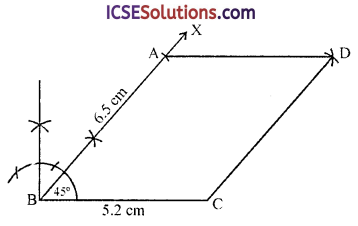Question 2.
Diagonals AC = 4.4 cm, diagonal BD = 6.8 cm and the angle between the diagonals = 60°.
Solution:
Steps of constructions :
(i) Draw diagonal BD = 6.8 cm and bisect it at O.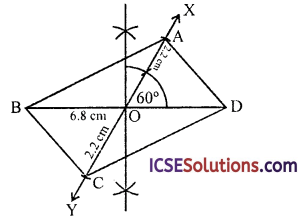(ii) From O, draw a ray OX making an angle of 60° and product it below.
(iii) Cut off OA = OC = $$\frac { 4.4 }{ 2 }$$ = 2.2 cm.
(iv) Join AB, BC, CD and DA.
ABCD is the required ||gm.

Question 3.
AB = 6 cm, BC = 4 cm and altitude = 2.9 cm.
Solution:
Steps of constructions :
(i) Draw a line segment AB = 6 cm.
(ii) At A, draw a perpendicular AX and cut off AP = 2.9 cm.(iii) From P, draw EPF || AB.
(iv) With centres B and A and radius 4 cm draw arcs cutting EF at D and C.
Then ABCD is the required ||gm.

Question 4.
AB = 4.3 cm, ∠B = 120° and the height = 3 cm.
Solution:
Steps of constructions :
(i) Draw a line segment AB = 4.3 cm.
(ii) At B, draw a ray BY.(iii) At A, draw perpendicular AX and cut off AE = 3 cm.
(iv) From E, draw a parallel line to AB which intersects BY at C.
(v) With centre A and radius BC, draw an arc cutting EF at D.
Then ABCD is the required ||gm.Question 5.
Using ruler and compasses only, construct a parallelogram ABCD using the following data: AB = 6 cm, AD = 3 cm and ∠DAB = 45°.
If the bisector of ∠DAB meets DC at P, prove that ∠APB is a right angle.
Solution:
Steps of constructions :
(i) Draw a line segment AB = 6 cm.
(ii) At A draw a ray AX making an angle of 45° and cut off AD = 3 cm.
(iii) With centres B and radius 3 cm and with centre D and radius 6 cm, draw arcs intersecting each other at C.
(iv) Join BC and DC.
ABCD is the required ||gm.
(v) Draw the angle bisector of A meeting CD at P and join BP.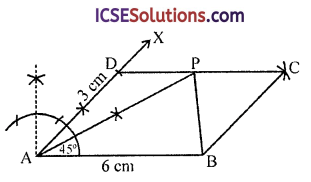To prove : ∠APB = 90°
∠DPA = ∠PAB (alternate angles)
But ∠DAP = ∠PAB (∵ AP is the bisector of CA)
∴ ∠DAP = ∠DPA = $$\frac { 134° }{ 2 }$$ = 67$$\frac { 1° }{ 2 }$$
∴ AD = DO = 3 cm
Similarly in ∆BCP
PC = DC – DP = 6 – 3 = 3 cm
∴ PC = CB = 3 cm
∴ ∠CPB = ∠CBP
But ∠CPB + ∠CBP = 180°- ∠BCP
= 180° – 45°= 135° (∵ ∠BCP = ∠DAB = 45°)
∴ ∠CPB = ∠CBP = $$\frac { 134° }{ 2 }$$ = 67$$\frac { 1° }{ 2 }$$
But ∠DPA + ∠CPB + ∠APB = 180°
(angles of a line)
⇒ 22$$\frac { 1° }{ 2 }$$ + 67$$\frac { 1° }{ 2 }$$ + ∠APB = 180°
⇒ 90° + ∠APB = 180°
⇒ ∠APB = 180° – 90° = 90°
Hence ∠APQ = 90°
Hence proved.

Question 6.
Using ruler and compasses construct a parallelogram ABCD given that AB = 4 cm, AC = 10 cm, BD = 6 cm and that AC and BD are the diagonals. Measure BC.
Solution:
Steps of constructions :
∵ The diagonals AC and BD of a ||gm ABCD bisect each other at O
∵ AO = OC = $$\frac { 10 }{ 2 }$$ = 5 cm 6
and BO = OD = $$\frac { 6 }{ 2 }$$ = 3 cm
(i) Draw a line segment AB = 4 cm.
(ii) With centre A and radius 5 cm and with centre B and radius 3 cm, draw arcs which intersect each other at O.
(iii) Join OA and OC.
(iv) Produce AO to C such that AO = OC and produce BO to B such that BO = OD
Then ABCD is the required ||gm.

Question 7.
Use ruler and compasses to construct a parallelogram with diagonals 6 cm and 8 cm in length having given the acute angle between them is 60°. Measure one of the longer sides.
Solution:
Steps of constructions :
(i) Draw a line segment AC = 10 cm and bisect it at O.
(ii) At O, draw a ray OX making an angle of 60° and produce XO to Y.
(iii) From O, cut off OD = OB = $$\frac { 6 }{ 2 }$$ = 3 cm.
(iv) Join AB, BC, CD and DA.
ABCD is the required ||gm.
Whose longer side is BC or AD, on measuring it, it is 6 cm long.Question 8.
The perpendicular distances between the pair of opposite sides of a parallelogram are 3 cm and 4 cm and one of its angles measures 60°. Using ruler and compasses only construct the parallelogram.
Solution:
Steps of constructions :
(i) Draw a line AX.
(ii) At A, draw a perpendicular and cut off AL = 3 cm.
(iii) Through L, draw LY parallel to AX.
(iv) From A, draw a ray making an angle of 60° which meets LY at D.
(v) At AD, draw a perpendicular and cut off AM = 4 cm.
(v) Through M, draw a line parallel to AD which meets LY at C.
Then ABCD is the required parallelogram.Question 9.
Using ruler and compasses only, draw a parallelogram whose diagonals are 5 cm and 7 cm long and which cut each other, so that one pair of the vertically opposite angles are each equal to 75°. Measure the length of the shorter side. State your steps of construction briefly.
Solution:
∵ Diagonals of a ||gm bisect each other
∴ AO = OC and OB = OD = 2.5 cm
Steps of constructions :
(i) Draw a line segment AC = 7 cm and bisect it at O.
(ii) At O, draw a line making an angle of 75° and produce it to both sides.
(iii) From O, cut off OB = OD = $$\frac { 5 }{ 2 }$$ = 2.5 cm.
(iv) Join AB, BC, CD and DA.
ABCD is the required ||gm.
Measuring its shorter side AD = 4 cm.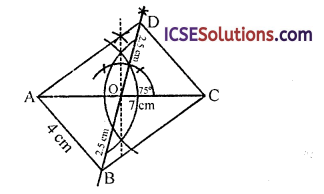Question 10.
Construct a rhombus ABCD on a base AB = 3.6 cm long and having one of its angles equal to 45°.
Solution:
Steps of constructions :
(i) Draw’ a line segment AB = 3.6 cm.
(ii) At A, draw a ray AX making an angle of 45° and cut off AB = 3.6 cm.
(iii) With centres B and D and radius 3.6 cm, draw arcs intersecting each other at C.
(iv) Join BC and CD.
Then ABCD is the required rhombus.Question 11.
Using ruler and compasses, draw a square on a line segment 3.2 cm long. Draw the diagonals. Measure their lengths and also the angle between their.
Solution:
Steps of constructions :
(i) Draw a line segment AB = 3.2 cm.
(ii) At Aand B, draw perpendiculars AX and BY and cut off AD = BC = 3.2 cm.
(iii) Join DC.
ABCD is the required square.
(iv) Join its diagonals AC and BD.
On measuring, each = 4.5 cm and each angle between these diagonals is 90° ∠AOB = 90°.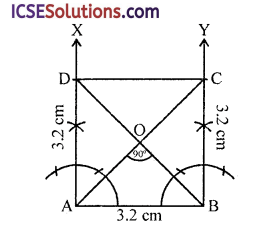Question 12.
Construct the square PQRS given the PR = 5 cm. Measure PQ.
Solution:
We know that diagonals of a square bisect each other at right angles and diagonals are also equal.
Steps of constructions :
(i) Draw a line segment. PR = 5 cm and draw its perpendicular bisector XY intersecting it at O.
(ii) Cut off OQ = OS = $$\frac { 5 }{ 2 }$$ = 2.5 cm.
(iii) Join PQ, QR, RS and SP respectively.
Then PQRS is the required square on measuring its side PQ which is = 3.6 cm.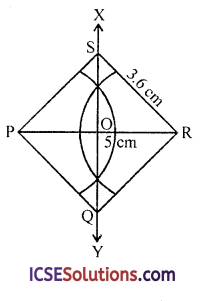Question 13.
Construct the rectangle whose sides are 2.5 cm and 4.3 cm.
Solution:
Steps of constructions :
(i) Draw a line segment AB = 4.2 cm.
(ii) At A and B, draw perpendiculars AX and BY and cut off AD = BC = 2.5 cm.
(iii) Join CD.
Then ABCD is the required rectangle.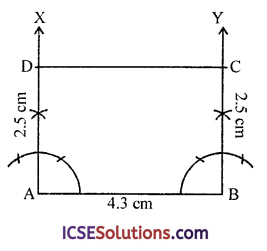Question 14.
Construct the rectangle ABCD given that diagonals intersect at an angle of 37° and that AC = 6 cm.
Solution:
Diagonals of a rectangle bisect each other and are equal in length.
Steps of constructions :
(i) Draw a line segment AC = 6 cm.
(ii) Bisect AC and O.
(iii) From O, draw a line XY.
Making an angle of 37° and produce it to both sides of O.
(iv) From XY cut off OC = OD = 3 cm.
(v) Join AB, BC, CD and AD.
Then ABCD is the required rectangle.Question 15.
Using ruler and compasses only, construct a square having a diagonal of length 5 cm. Measure its side correct to the nearest millimetre
Solution:
The diagonals of a square bisect each other at right angles and are also equal in length. Steps of constructions:
(i) Draw a line segment AC = 5 cm.
(ii) Draw its perpendicular bisector XY intersecting it at O.
(iii) Cut off OB = OD = 2.5 cm.
(iv) Join AB, BC, CD and DA respectively.
Then ABCD is the required square.
On measuring its side, it is 3.6 cm each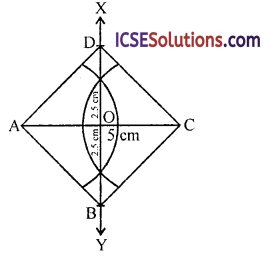Question 16.
Using ruler and compasses only construct a rectangle each of whose diagonals measures 6 cm and the diagonals intersect at an angle of 45°.
Solution:
Diagonals of rectangle bisect each other and are equal to each other in length.
Steps of constructions :
(i) Draw a line segment AC = 6 cm and bisect it at O.
(ii) At O, draw a line XY making an angle of 45° and produce it to both sides of O.
(iii) Cut off OB = OD = $$\frac { 6 }{ 2 }$$ = 3 cm.
(iv) Join AB, BC, CD and DA respectively.
Then ABCD is the required rectangle.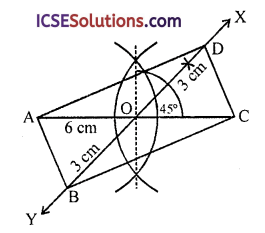Question 17.
Construct a rhombus PQRS whose diagonals PR, QS are 8 cm and 6 cm respectively. Find by construction a point X equidistant from PQ, PS and equidistant from R, S. Measure XR.
Solution:
Diagonals of a rhombus bisect each other at right angles.
Steps of constructions :
(i) Draw a line segment PR = 8 cm.

(ii) Draw its perpendicular bisector XY which intersects PR at O.

(iii) From XY, cut off OQ = OS = $$\frac { 6 }{ 2 }$$ = 3 cm.

(iv) Join PQ, QR, RS and SP.
Then PQRS is the required rhombus.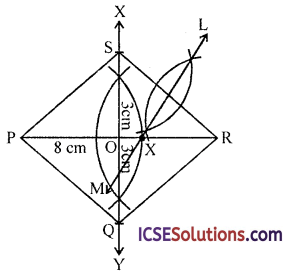(v) ∵ The required point X is equidistant from P and PS.
∴ It lies on the angle bisector of ∠QPS which is the diagonal PR
∵ It is equidistant from R and S
Then it will be on the perpendicular bisector of RS
Now draw perpendicular bisector of RS which intersects PR at X
Then X is the required point.
Construct the trapezium PQRS in which PQ is parallel to RS, from the given measurements in problem 18 to 20, without using set squares and protractor as far as possible.Question 18.
PQ = 7 cm, QR = 3.5 cm, PS = 2.5 cm, SP = 3 cm.
Solution:
Steps of constructions :
(i) Draw a line segment PQ = 7 cm.
(ii) Take a point E such that AE = SR = 2.5 cm and then EQ = 7 – 2.5 = 4.5 cm.
(iii) With centre E and radius 3 cm and with centre Q and radius 3.5 cm draw arcs which intersect each other at R.
(iv) Join ER and QR.
(v) With centre R and radius 2.5 cm and with centre P and radius 3 cm, draw arcs intersecting each other at S.
(vi) Join RS and PS.
Then PQRS is the required trapezium.Question 19.
PQ = 8 cm, QR = 4 cm, RS = 3 cm, SP = 2 cm. Measure ∠QPS.
Solution:
Steps of constructions :
(i) Draw a line segment PQ = 8 cm.
(ii) Cut off PE = RS = 3 cm.
(iii) With centre E and radius 2 cm and with centre Q and radius 4 cm draw arcs intersecting each other at R.
(iv) Join ER and QR.
(v) With centre P and radius 2 cm and with centre R and radius 3 cm, draw arcs intersecting each other at S.
(vi) Join PS and SR.
Then PQRS is the required trapezium.
On measuring the angle QPS, it is 50°.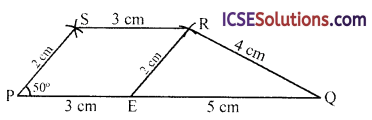Question 20.
Without using set squares or protractor, construct the trapezium ABCD in which AB is parallel to DC, AB = 4.0 cm, ∠ABC = 120°, BC = 2.5 cm, DA = 2.4 cm and ∠DAB is obtuse, Measure AC.
Solution:
∵ ∠ABC = 120° and AB || DC
∴ ∠BCD = 180°- 120° = 60°
(Cointerior angle)
(i) Draw a line segment AB = 4 cm.
(ii) At B, draw a ray BX making an angle of 120° and cut off BC = 2.5 cm.
(iii) At C, draw and the ray CY making an angle of 60°.
(iv) With centre A and radius 2.4 cm draw an arc whcih intersects CY at D’ and D
∵ ∠A is obtuse and by joining AD’, then angle at A will be acute
Then ABCD is the required trapezium on measuring AC, it is 5.5 cm.Question 21.
Construct the trapezium ABCD in which AB is parallel to DC. AB = 6.5 cm, CD = 3 cm, AC = 7 cm and DB = 5 cm.
Solution:
Steps of constructions :
(i) Draw a line segment AB = 6.5 cm.
(ii) Produce it to P such that BP = 3 cm.
(iii) With centre P and radius 5 cm and with centre A and radius 7 cm draw arcs which intersect each other at C.
(iv) Join AC and PC and BC.
(v) With centre B and radius 5 cm and with centre C and radius 3 cm, draw arcs intersecting eachother at D.
(vi) Join AD, BD and CD.
Then ABCD is the required trapezium.Question 22.
Hexagon on a side of 4 cm. Find out its area.
Solution:
Steps of constructions :
The radius of the circumcircle of a regular hexagon is the length of the side of the regular hexagon.
(i) Draw a circle with centre O and radius 4 cm.
(ii) Take a point A on it and from A, draw arcs of 4 cm cutting the circle at B, C, D, E and F.
(iii) Join AB, BC, CD, DE, EF and FA.Then ABCDEF is the required regular hexagon
The area of regular hexagon = 6 x $$\frac{\sqrt{3}}{4}$$ (side)²
= $$\frac{6 \sqrt{3}}{4}$$ (4)²
= $$\frac{6 \times \sqrt{3} \times 16}{4}$$
= 24 (1.732) cm²
= 41.568 cm²

Question 23.
Hexagon on a side of 3.2 cm. Find out its area.
Solution:
Steps of constructions :
The radius of the circumcircle of a regular hexagon is the length of the side of this hexagon.
(i) Draw a circle with centre O and radius 3.2 cm.
(ii) Take a point A on the circle.
(iii) Start from A and radius 3.2 cm the arcs on the circle at B, C, D, E and F.
(iv) Join AB, BC, CD, DE, EF and FA.
Then ABCDEF is the required regular hexagon.